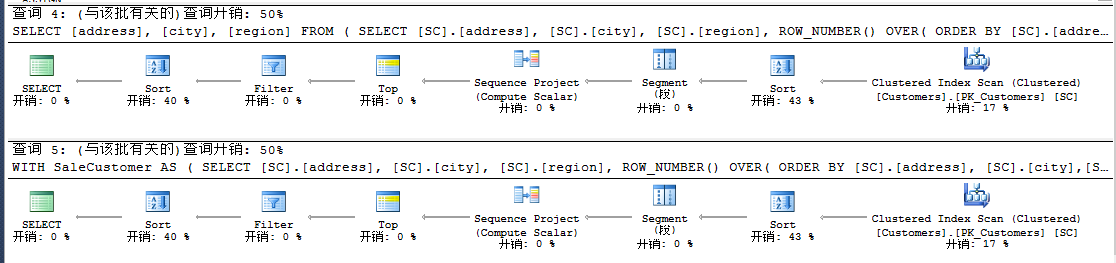`  USE TSQL2012  GO  DECLARE @StartRow INT  DECLARE @EndRow INT  SET @StartRow = 31  SET @EndRow = 40  SELECT [address], [city], [region]  FROM (  SELECT [SC].[address], [SC].[city], [SC].[region],  ROW_NUMBER() OVER(  ORDER BY [SC].[address], [SC].[city],[SC].[custid]) AS RowNumber  FROM Sales.Customers SC) SaleCustomer  WHERE RowNumber > @StartRow AND RowNumber < @EndRow  ORDER BY [address], [city], [region];`

`  WITH SaleCustomer AS  (  SELECT [SC].[address], [SC].[city], [SC].[region],  ROW_NUMBER() OVER(  ORDER BY [SC].[address], [SC].[city],[SC].[custid]) AS RowNumber  FROM Sales.Customers SC)  SELECT [address], [city], [region]  FROM SaleCustomer  WHERE RowNumber > @StartRow AND RowNumber < @EndRow  ORDER BY [address], [city], [region]  GO``  USE TSQL2012  GO  DECLARE @PageSize INT = 10, @PageIndex INT = 3  SELECT *  FROM Sales.Customers  ORDER BY custid  OFFSET @PageIndex * @PageSize ROWS  FETCH NEXT 10 ROWS ONLY  GO`

COALESCE compare to ISNULL

`  USE TSQL2012  GO  SELECT custid, country, region, city,   country + COALESCE(N''+ region, N'') + N',' + city AS location  FROM Sales.Customers``  USE TSQL2012  GO  SELECT custid, country, region, city,  country + CONCAT(country,N''+ region, N',' + city) AS location  FROM Sales.Customers`msdn对CONCAT函数解释为：CONCAT 采用可变数量的字符串参数，并将它们串联成单个字符串。 它需要至少两个输入值；否则将引发错误。 所有参数都隐式转换为字符串类型，然后串联在一起。 Null 值被隐式转换为空字符串。 如果所有参数都为 Null，则返回 varchar(1) 类型的空字符串。 隐式转换为字符串的过程遵循现有的数据类型转换规则。

COALESCE与ISNULL函数探讨

（1）COALESCE和ISNULL处理数据类型优先不同

COALESCE函数决定类型输出基于数据类型优先【data type precedence】，所以如下在处理INT时，DATETIME优先级高于INT。

`  DECLARE @int INT, @datetime DATETIME;  SELECT COALESCE(@datetime, 0);  SELECT COALESCE(@int, CURRENT_TIMESTAMP);``  DECLARE @int INT, @datetime DATETIME;  SELECT ISNULL(@datetime, 0);``  DECLARE @int INT, @datetime DATETIME;  SELECT ISNULL(@int, CURRENT_TIMESTAMP);``  DECLARE @int INT, @datetime DATETIME;  SELECT ISNULL(@int, CONVERT(INT,CURRENT_TIMESTAMP));  SELECT ISNULL(@int, CAST(CURRENT_TIMESTAMP AS INT));`

（2）ISNULL会造成数据丢失

`  DECLARE @c5 VARCHAR(5);  SELECT 'COALESCE', COALESCE(@c5, 'Jeffcky Wang')  UNION ALL  SELECT 'ISNULL', ISNULL(@c5, 'Jeffcky Wang');``  DECLARE @c5 VARCHAR(5);  SELECT    c = COALESCE(@c5, 'Jeffcky Wang'),    i = ISNULL(@c5, 'Jeffcky Wang')  INTO dbo.TestISNULL_COALESCE  SELECT name, t = TYPE_NAME(system_type_id), max_length, is_nullable   FROM sys.columns   WHERE [object_id] = OBJECT_ID('dbo.TestISNULL_COALESCE');`（3）COALESCE对列计算时需要持久化

`  CREATE TABLE dbo.CreateISNULL  (   a INT,   b AS ISNULL(a, 15) PRIMARY KEY  );``  CREATE TABLE dbo.CreateCOALESCE  (   a INT,   b AS COALESCE(a, 15) PRIMARY KEY  );``  CREATE TABLE dbo.CreateCOALESCE  (   a INT,   b AS COALESCE(a, 15) PERSISTED PRIMARY KEY  );`

`  DECLARE @c CHAR(10);  SELECT 'x' + COALESCE(@c, '') + 'y';  SELECT 'x' + ISNULL(@c, '') + 'y';`（4）COALESCE函数支持超过两个参数

`SELECT COALESCE(a, b, c, d, e, f, g) FROM dbo.table;`

`SELECT ISNULL(a, ISNULL(b, ISNULL(c, ISNULL(d, ISNULL(e, ISNULL(f, g)))))) FROM dbo.table;`

`  CASE   WHEN [tempdb].[dbo].[table].[a] IS NOT NULL THEN [tempdb].[dbo].[table].[a]    ELSE CASE WHEN [tempdb].[dbo].[table].[b] IS NOT NULL THEN [tempdb].[dbo].[table].[b]    ELSE CASE WHEN [tempdb].[dbo].[table].[c] IS NOT NULL THEN [tempdb].[dbo].[table].[c]    ELSE CASE WHEN [tempdb].[dbo].[table].[d] IS NOT NULL THEN [tempdb].[dbo].[table].[d]    ELSE CASE WHEN [tempdb].[dbo].[table].[e] IS NOT NULL THEN [tempdb].[dbo].[table].[e]    ELSE CASE WHEN [tempdb].[dbo].[table].[f] IS NOT NULL THEN [tempdb].[dbo].[table].[f]    ELSE [tempdb].[dbo].[table].[g] END END END END END END`

（5）COALESCE和ISNULL二者性能比较

`  DBCC DROPCLEANBUFFERS;  DECLARE    @a VARCHAR(5), -- = 'str_a', -- this line changed per test   @b VARCHAR(5), -- = 'str_b', -- this line changed per test   @v VARCHAR(5),    @x INT   = 0,   @time DATETIME2(7) = SYSDATETIME();  WHILE @x <= 500000  BEGIN   SET @v = COALESCE(@a, @b); --COALESCE   SET @x += 1;  END  SELECT DATEDIFF(MILLISECOND, @time, SYSDATETIME());  GO  DBCC DROPCLEANBUFFERS;  DECLARE    @a VARCHAR(5), -- = 'str_a', -- this line changed per test   @b VARCHAR(5), -- = 'str_b', -- this line changed per test   @v VARCHAR(5),    @x INT   = 0,   @time DATETIME2(7) = SYSDATETIME();  WHILE @x <= 500000  BEGIN   SET @v = ISNULL(@a, @b); --ISNULL   SET @x += 1;  END  SELECT DATEDIFF(MILLISECOND, @time, SYSDATETIME());``  SELECT COALESCE((SELECT MAX(index_id) FROM sys.indexes WHERE [object_id] = t.[object_id]), 0)   FROM sys.tables AS t;  SELECT ISNULL((SELECT MAX(index_id) FROM sys.indexes WHERE [object_id] = t.[object_id]), 0)   FROM sys.tables AS t;`（6）ISNULL和自然语言描述不一致

`  IF ISNULL(something)   -- do something`

`  IF something IS NULL   -- do something  -- or  IF ISNULL(something, NULL) IS NULL   -- do something  -- or  IF ISNULL(something, '') = ''   -- do something`

（7）利用GUID看看奇葩的ISNULL

`SELECT ISNULL(NEWID(), 'JeffckyWang') AS Col1``  SELECT ISNULL(NEWID(), 'JeffckyWang') AS Col1  INTO dbo.IsNullExample2;  EXEC sp_help 'dbo.IsNullExample2';``  SELECT    ISNULL(argument, '')   INTO dbo.IsNullExample;`